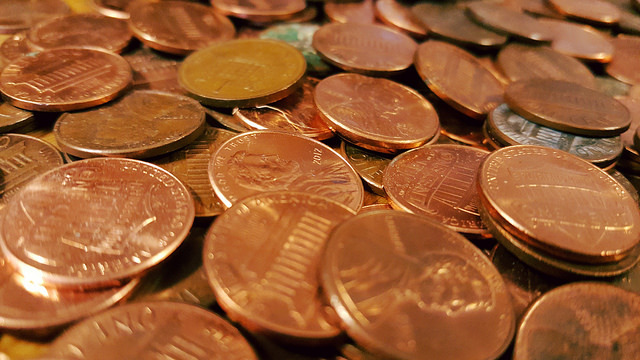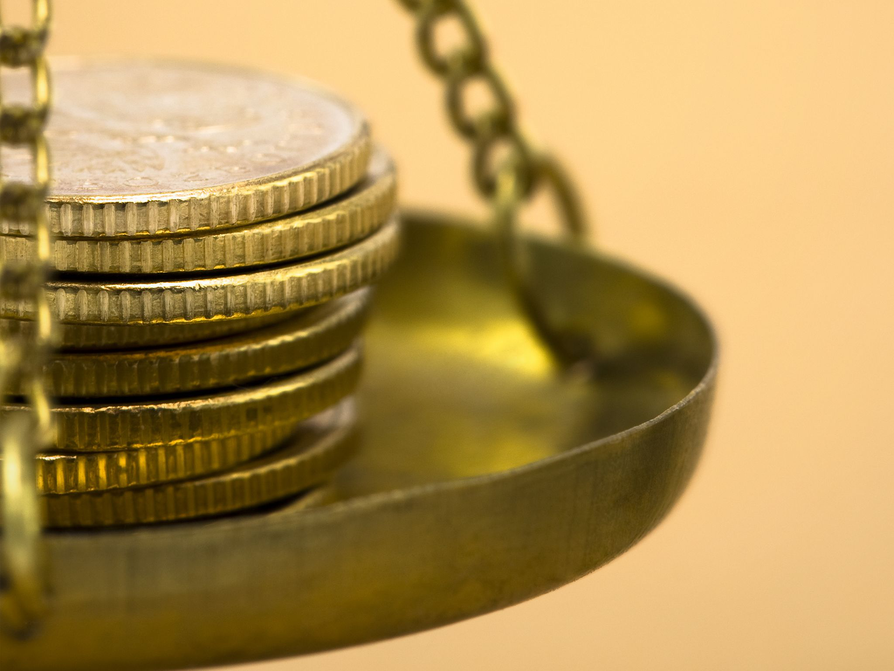Subject:
Numbers and Operations
Material Type:
Lesson Plan
Level:
Middle School
6
Provider:
Pearson
Tags:
• Inequality
• Number Line
Language:
English
Media Formats:
Interactive, Text/HTML

# Balance Scale As A Mathematical Model# Lesson Overview

Students solve a classic puzzle about finding a counterfeit coin. The puzzle introduces students to the idea of a scale being balanced when the weight of the objects on both sides is the same and the scale being unbalanced when the objects on one side do not weigh the same as the objects on the other side.

# Key Concepts

The concept of an inequality statement can be modeled using an unbalanced scale. The context—weighing a set of coins in order to identify the one coin that weighs less than the others—allows students to manipulate the weight on either side of the scale. In doing so, they are focused on the relationship between two weights—two quantities—and whether or not they are equal.

# Goals and Learning Objectives

• Explore a balance scale as a model for an equation or an inequality.
• Introduce formal meanings of equality and inequality.

# Lesson Guide

Present the situation and help students understand it as needed. Have students look at the Hint if they do not know the meaning of counterfeit.

Have students turn and talk to their partners about their ideas for using the balance scale to identify the counterfeit coin. Be sure that students understand that they can put more than one coin on each side of the scale at a time.

SWD: Help students with disabilities build their mathematical vocabulary by continually modeling the use of new terms in the context of classroom work and activities.

# Teacher Demo

Make time to demonstrate how a real balance scale works. Show students how to use the balance scale to compare weights.

# Which Coin Is Counterfeit?

Suppose you have 12 coins, and one of the coins is counterfeit. You know that the counterfeit coin weighs less than the other coins.

1. Can you use a balance scale to find the counterfeit coin?
2. Turn and talk to your partner about this question. Share an idea about how you might approach the problem, but do not try to find the solution yet.## Hint:

• Counterfeit means fake ; a counterfeit coin looks like a real coin issued by the government but is actually an imitation.
• A counterfeit coin is made from cheaper metals, so it weighs less than a real coin.

# Lesson Guide

Discuss the Math Mission. Students will develop a strategy for consistently finding the counterfeit coin in a group of real coins using a balance scale.

## Opening

Develop a strategy for consistently finding the counterfeit coin in a group of real coins using a balance scale.

# Lesson Guide

Circulate around the room. Remind students that they should describe their methods in writing.

# Preparing for Ways of Thinking

Listen and look for the following ways of thinking, and identify students whose work would be good for the whole-class discussion.

Look for students who:

• Remain in guess-and-check mode. (Let these students play with different ideas, but ask them to record one or two elements of a strategy that they think will work.)
• Restrict themselves to placing only 1 coin at a time on each side of the balance. (This approach will work if done systematically, and it might be interesting for the class to hear from students about how the strategy did or did not work.)
• Divide the coins into 2 groups of 6 coins, placing each group on one side of the scale to identify the lighter side and then repeating with the lighter group.
• Divide the coins into 4 groups of 3 coins, placing each group on one side of the scale to identify the lighter side and then figuring out which of the 3 remaining coins is lighter by placing pairs of coins on the scale in turns.
• Start with 1 coin on each side of the scale and add a single coin to each side systematically until the scale is unbalanced, which signals the presence of the counterfeit coin.
• Divide the coins into 3 groups of 4 coins, placing 1 group of 4 coins on either side of the scale. If the scale is balanced, the students recognize that the coin is among the third group of 4 coins not on the scale. Students then divide the group with the counterfeit coin into 2 groups and continue dividing the group with the counterfeit coin into 2 groups until they have identified the counterfeit coin.
• Represent the situation using numbers, symbols, and letters in equations and inequalities from the start, and who record what the numbers and letters represent.

SWD: Students with disabilities may struggle to self-monitor whether strategies are effective or ineffective. Help students to reflect by modeling think-aloud strategies, if students have difficulty changing approaches from an ineffective strategy.

ELL: Allow students time to explain how they are doing what they are doing if it is not readily apparent when you observe.

# Mathematical Practices

Mathematical Practice 1: Make sense of problems and persevere in solving them.

Students must make sense of the situation in order to find the counterfeit coin. Look for different methods of solving the problem, and identify students to share their strategies.

Mathematical Practice 2: Reason abstractly and quantitatively.

Look for students who use symbols (=, <, and >) to represent the situation. These students are able to decontextualize the situation and represent it symbolically.

# Interventions

Student does not recognize that the information he is looking for is whether or not the scale is balanced.

• What does it mean if the scale is balanced?
• What does it mean if the scale is unbalanced?
• If the scale is unbalanced, do you know something about where the counterfeit coin is?

Student may not realize that the heavier pan will be lower (not higher) than the other pan.

• Think about putting a feather and a watermelon on the scale. Which side will be lower?

Student realizes that splitting the coins into groups is a good strategy but does not know where to go from there.

• Try dividing the coins into 2 groups. What does the scale tell you?
• Try dividing the coins into 3 groups. What does the scale tell you?
• Once you know you have a real coin, use it to gain information about the other coins.

Student has trouble keeping track of coins already weighed or keeping track of information found in the process of solving the problem.

• Can you organize the information you have gathered in a table or a diagram?
• Can you write equations and inequality statements to keep track of what you know?

See the possible approaches described in Preparing for Ways of Thinking. Students must write their strategies down. Here are two approaches that are within reason at this level (neither solves the problem in the fewest steps possible):

Approach 1

• Let a represent the weight of a genuine coin and let b represent the weight of the counterfeit coin.
• Split the coins into 2 groups of 6, and compare the weight of each group.
6a > 5a + b
• Split the right side into 2 groups of 3 coins and compare the weight of each group.
3a > 2a + b
• Compare the weights of pairs of single coins a1a2, and b in turn, until the scale does not balance:
• Possibility 1: a1 = a2
• Possibility 2 (and Step 2 after Possibility 1): a1 > b and a2 > b

Approach 2

Place a single coin on each side of the scale, and then add a single coin to each side in turn until the scale is not balanced. (The unbalanced scale is shown in the final step here, but this could happen at any of the earlier steps.)

$\begin{array}{c}a=a\\ a+a=a+a\\ a+a+a=a+a+a\\ a+a+a+a=a+a+a+a\\ a+a+a+a+a=a+a+a+a+a\\ a+a+a+a+a+a>a+a+a+a+a+b\end{array}$

Some students may notice that the final inequality is equivalent to the inequality used to model the first step in the previous approach: 6a > 5a + b.

# Challenge Problem

• Strategies will vary.
• Check students' work in the interactive.

# Develop a Strategy

Think back to the problem you just discussed:

Suppose you have 12 coins, and one of the coins is counterfeit. You have a balance scale, and you know that the counterfeit coin weighs less than the other coins.

• Using the Balance Scale Game interactive, develop a strategy for finding the counterfeit coin.

# Challenge Problem

In another group of 12 coins, there are 2 counterfeit coins that are lighter than the rest.

• How will you have to adjust your strategy to find the 2 counterfeit coins?
• Use the Balance Scale interactive to find the 2 counterfeit coins.

INTERACTIVE: Balance Scale Game

## Hint:

Suppose you have 6 coins on each side of the balance scale and the left side is heavier. What does this situation tell you?

# Lesson Guide

Both sides of the balance must be considered at the same time to gain any information about the coins.

Bring out the following points as students share their strategies:

• An equation, which includes the equal sign (=), can be written to represent any balance of weights on the scale.
• An inequality statement, which includes the < symbol (is less than) or the > symbol (is greater than), can be written to represent an unbalanced scale.
• When the scale is balanced, this means the weight on one side is equal to the weight on the other side.
• When the scale is not balanced, this means the weight on one side is greater than or less than the weight on the other side.
• When you add or remove weights from one side, the balance shifts accordingly.
• When a scale remains balanced after you have removed or added something from both sides, it means you have added or removed an equal amount from both sides.

Focus on the correct usage of the = sign and the < and > symbols in students' shared work.

Highlight student talk that clarifies the meaning of a balanced scale and an unbalanced scale, and how to represent balanced and unbalanced mathematically in words and symbols.

Draw attention to how students are using the terms equation and inequality in their explanations, and relate these terms as needed to uses of equalbalancedunequalunbalanced, and any other terms students use to describe the equality or lack of equality between the sides of the scale.

• How can you represent a situation symbolically if one side is heavier than another?
• How can you represent a situation symbolically if both sides are equal?
• What strategies did you use in solving the problem?
• Compare [Name]'s strategy and [Name]'s strategy:
• How are they similar?
• How are they different?
• Whose strategy makes more sense to you? Why?

ELL: Speak clearly and monitor your speech for slang and idiomatic expressions. Use intonation, volume, and pauses to help make your meaning clear. Rephrase instead of repeating; paraphrase important concepts and directions.

# Ways of Thinking: Make Connections

Take notes about the different strategies your classmates used for finding the counterfeit coin(s).

## Hint:

• For one counterfeit coin: How do you know which side of the balance scale the counterfeit coin is on?
• For two counterfeit coins: How can you be sure that both of the counterfeit coins are on that side of the balance scale?